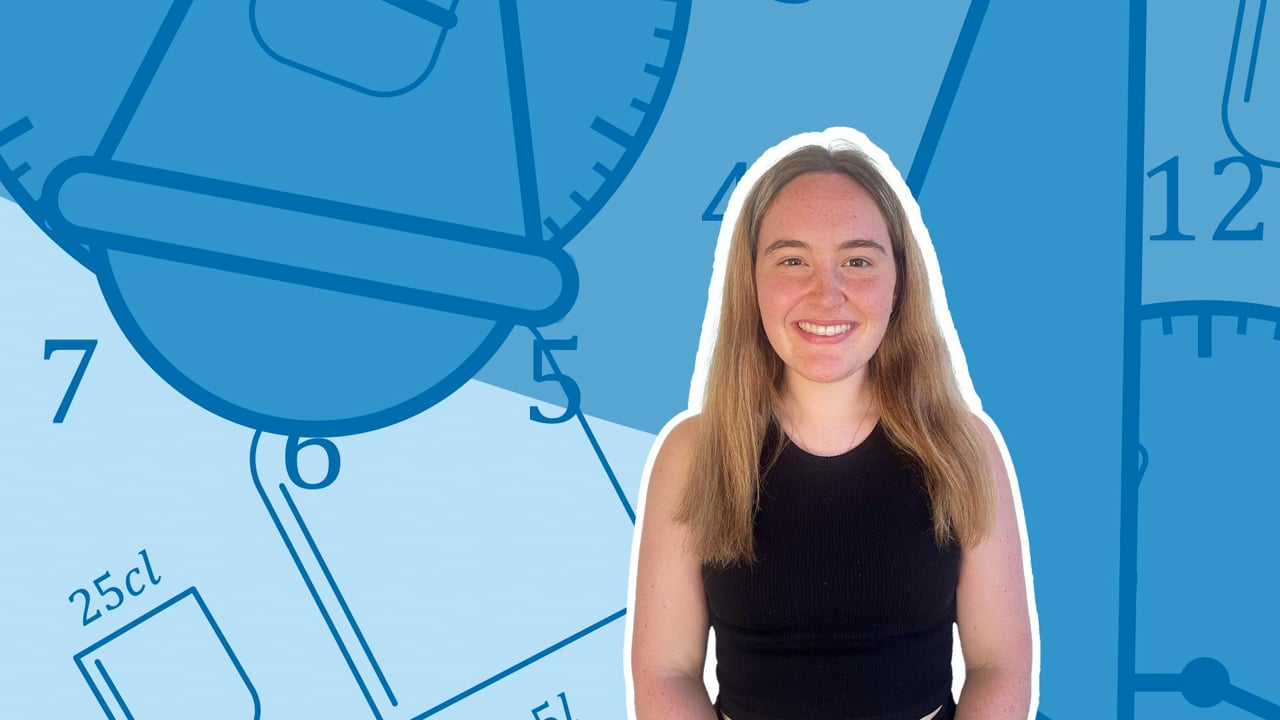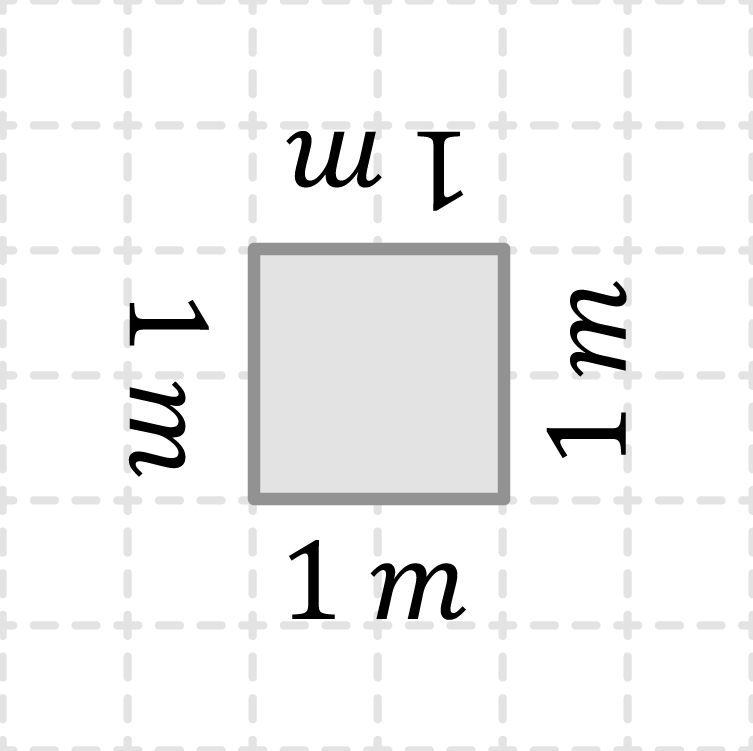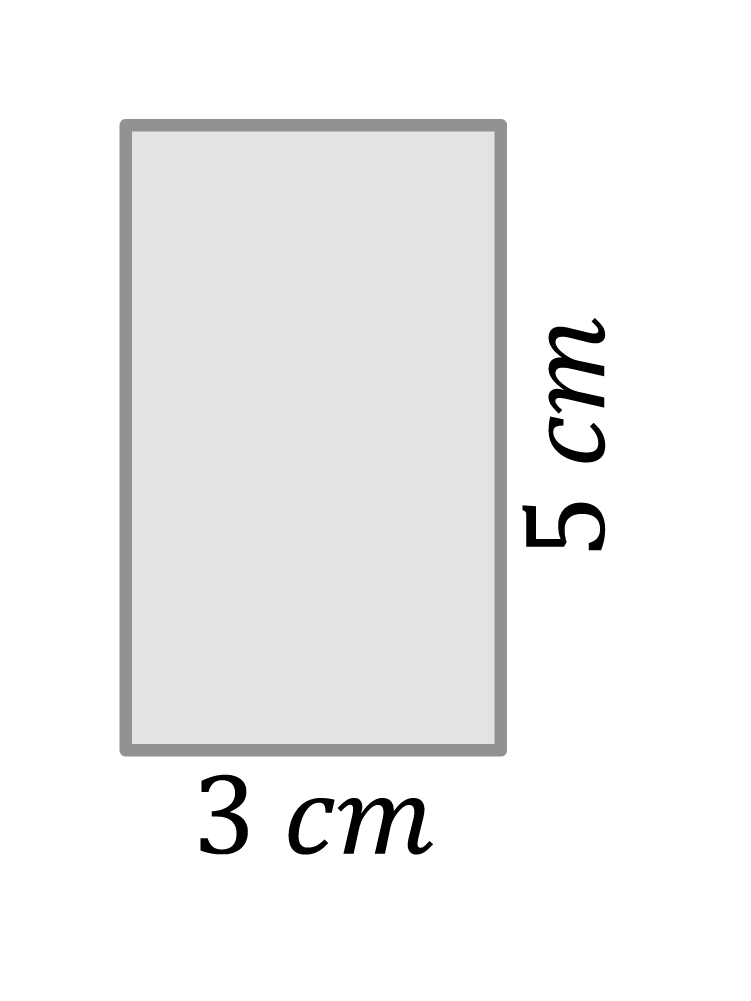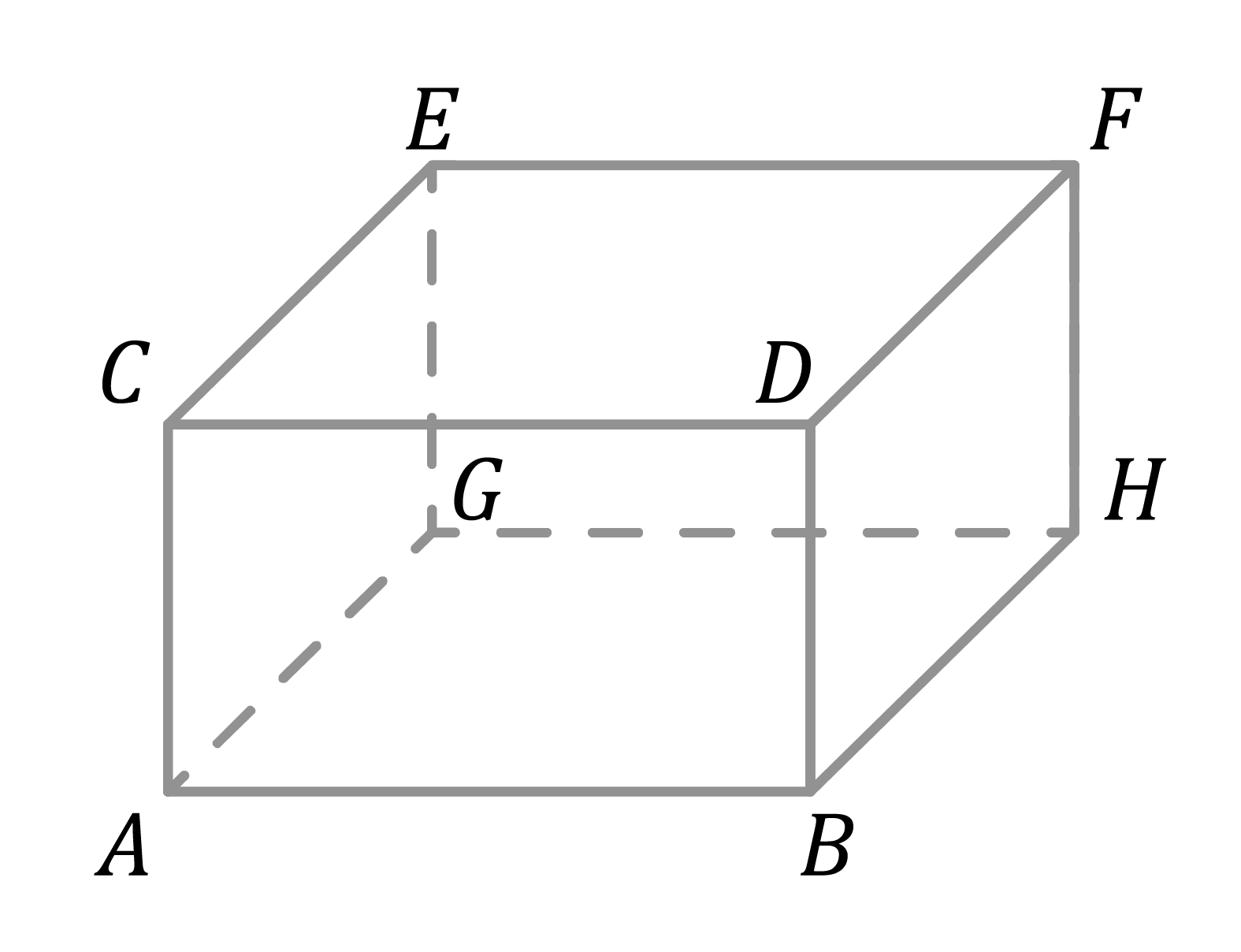Chapter OverviewMaths

Exam board

Pearson Edexcel

Number

Algebra

Graphs

Ratio proportion and rates of change

Shapes and area

Angles and geometry

Trigonometry

Probability

Statistics

Maths

# Converting units: area and volume0%

Summary

# Converting units: area and volume

## ​​In a nutshell

Area and volume unit conversions are almost identical to regular unit conversions except for one thing: they involve multiply or dividing by the conversion factor more than once.

## Area unit conversions

When converting between units of area, the steps are very similar to converting with regular lengths. However, since area is measured in units squared, multiplying and dividing by the conversion factor has to happen twice.

#### PROCEDURE

 1 Identify the conversion factor. 2 Decide whether to divide or multiply. 3 Divide or multiply by the conversion factor twice.

Tip: Remember the units in your answer should be squared (followed by a $^2$).

##### Example 1

Take a look at the $1m \times 1m$ cube. What is its area in metres squared?

 Work out the area in metres squared.$1\times1=1m^2$Identify the conversion factor.$1m=100cm$Conversion factor = $100$​​Multiply by the conversion factor twice to go from metres squared (big) to centimetres squared (small).$1m^2\times100\times100=\underline{10,000cm^2}$​​​##### Example 2

What is the area of the rectangle? Give your answer in metres squared.

 Work out the area in centimetres squared.$3\times5=15cm^2$Identify the conversion factor.$1m=100cm$Conversion factor $=100$​​​Divide by the conversion factor twice to go from centimetres squared (small) to metres squared (big).$5cm^2\div100\div100=\underline{0.0015m^2}$​​## Volume unit conversions

Volume unit conversions are treated in the same way as area conversions except that instead of multiplying/dividing twice, it happens three times.

#### PROCEDURE

 1 Identify the conversion factor. 2 Decide whether to divide or multiply. 3 Divide or multiply by the conversion factor three times.

Tip: Remember the units in your answer should be cubed (followed by a $^3$).

##### Example 3

The cuboid shown has a volume of $2.5m^3$. What is its volume in centimetres cubed?​

 Work out the conversion factor.$1m=100cm$Conversion factor $=100$​Multiply by the conversion factor three times to go from metres cubed (big) to centimetres cubed (small).$2.5m^3\times100\times100\times100=\underline{2,500,000cm^3}$​## Want to find out more? Check out these other lessons!

Formulae for the area and volume of shapes

FAQs

• Question: How do I convert between units of volume?

Answer: To convert between units of volume: 1. Identify the conversion factor. 2. Decide whether to divide or multiply. 3. Divide or multiply by the conversion factor three times.

• Question: How do I convert between units of area?

Answer: To convert between units of area: 1. Identify the conversion factor. 2. Decide whether to divide or multiply. 3. Divide or multiply by the conversion factor twice.

Theory

Exercises